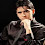# Mental Maths (Subtractions)In this post, we will show you how to do Subtractions Mentally. Have a look at the following examples....

### 987 - 256 = ?

• Instead of taking a single digit at a time, subtractions would be faster by taking two digits.... See here
• 87 - 56 = 31
• 900 - 200 = 700
• So, the result of 987 - 256 = 731

### 824 - 587 = ?

• Take 100s Complement of 87 (i.e. 100 - 87) which is 13 and add it to 24. The result is 37. This gives the units and tens digits of the result. Since 24 < 87, we have actually subtracted 87 from 124 i.e. we have borrowed 1 from 8 ( of 824). So, we now do (7-5) = 2. The result is 237. (For new learners, this technique may look lil confusing. If you feel it is confusing, then just read it for two times and try to understand the logic behind it).

### 9217 - 858 = ?

• Adding 100s complement of 58 (which is 42, i mean 58 + 42 = 100) to 17, we get (42+17) = 59 which gives the units and 10s digits of the result.
• Since 58 is greater than 17, we have to borrow 1 from 92 which leaves us with 91.  So, the first part of the answer is 91 - 8 = 83. SO, the result is 8359.

### 934 - 286 + 847 - 798 = ?

• When we have a combination of additions and subtractions, first add all the numbers with + sign before them and add all the numbers with - sign before them.
• I mean (934 + 847)  - (286 + 798) = 1781 - 1084
• By applying the method explained in previous examples, 1781 - 1084 = 697.

1.awesome technique thanks a lot

2.Good shortcut !! :)

3.4.5.Preeti :
hmm.. cool

6.Thank U.
I guess this is vedic maths... We'd be happier if u'd provide some more Vedic Math material... it makes the stuff easier n faster.

7.where is the mental addition page?

8.Great Job Sir

9.great one....easy to remember and calculate

10.Nice Treatment
ttt

11.very nice formula for quick solution

12.I never knew this technique :) :) :)